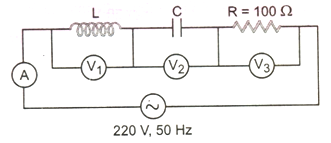# Alternating Currents

15 QuestionsSettingsTime: 30 Minute

Related Topics
• 1.
If the rms current in a 50 Hz ac circuit is 5 A, the value of the current 1/300 seconds after its value becomes zero is
• A.
• B.
• C.
• D.
• 2.
To reduce the reasonant frequency in an LCR series circuit with a generator
• A.

The generator frequency should be reduced

• B.

Another capacitor should be added in parallel to the first

• C.

The iron core of the inductor should be removed

• D.

Dielectric in the capacitor should be removed

• 3.
An inductor of reactance 1 Ω and a resistor of 2 Ω are connected in series to the terminals of a 6 V (rms) a.c. source. The power dissipated in the circuit is
• A.

8 W

• B.

12 W

• C.

14.4 W

• D.

18 W

• 4.
The natural frequency of an LC circuit is 1, 25, 000 c/s. Then the capacitor C is replaced by another capacitor with a dielectric medium of constant K. In this case, the frequency decreases by 25 kHz. The value of K is
• A.

1.56

• B.

2.1

• C.

3

• D.

1.7

• 5.
In the given circuit: The reading of voltmeter V1 and V2 are 300 volts each. The reading of the voltmeter V3 and ammeter A are respectively
• A.

150 V, 2.2 A

• B.

220 V, 2.2 A

• C.

220 V, 2.0 A

• D.

100 V, 2.0 A

• 6.
In the circuit: What is the energy stored in the coil at steady state?
• A.

21.3 J

• B.

42.6 J

• C.

Zero

• D.

213 J

• 7.
• A.

50 W

• B.

100 W

• C.

200 W

• D.

400 W

• 8.
A pure resistive circuit element X when connected to an ac supply of peak voltage 200 V gives a peak current of 5 A which is in phase with the voltage. A second circuit element Y, when connected to the same AC supply also gives the same value of peak current but the current lags behind by 90 o. If the series combination of X and Y is connected to the same supply, what will be the rms value of current?
• A.
• B.
• C.
• D.
• 9.
For the LR circuit: The phase angle if frequency is 100/π is
• A.

30 o

• B.

60 o

• C.

45 o

• D.

90 o

• 10.
An AC voltage source of variable angular frequency ω and fixed amplitude V0 is connected in series with a capacitance C and an electric bulb of resistance R (inductance zero). When ω is increased
• A.

The bulb glows dimmer

• B.

The bulb glows brighter

• C.

Total impedance of the circuit is unchanged

• D.

Total impedance of the circuit increases

• 11.
In a series LCR circuit R = 200 Ω and the voltage and the frequency of the main supply is 220 V and 50 Hz respectively. On taking out the capacitance from the circuit, the current lags behind the voltage by 30o. On taking out the inductor from the circuit, the current leads the voltage by 30o. The power dissipated in the LCR circuit is
• A.

210 W

• B.

Zero W

• C.

242 W

• D.

305 W

• 12.
An ac voltage is applied to a resistance R and an inductor L in series. If R and the inductive reactance are both equal to 3 Ω, the phase difference between the applied voltage and the current in the circuit is
• A.

π/6

• B.

π/4

• C.

π/2

• D.

Zero

• 13.
If an electrical circuit R, L, C and an a.c. voltage source are all connected in series. When L is removed from the circuit, the phase difference between the voltage and the current in the circuit is π/3. If instead, C is removed from the circuit, the phase difference is again π/3. The power factor of the circuit is:
• A.
• B.
• C.
• D.
• 14.
An LCR series circuit is connected to a source of alternating current. At resonance, the applied voltage and the current flowing through the circuit will have a phase difference of
• A.

π

• B.

π/2

• C.

π/4

• D.

0

• 15.
The electrical energy spent in running the circuit for one hour is
• A.

20.05 J

• B.

20.05 k w h

• C.

7.22 x 104 J

• D.

Zero